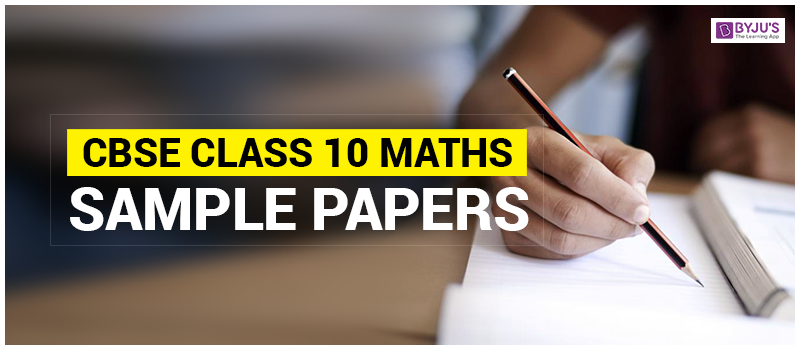# CBSE Class 10 Maths Sample Papers 2020 - Download Basic and Standard Paper

CBSE Sample Paper for Class 10 Maths the best resources to prepare for your board exam. Here our Maths experts have made sample Papers available for students so they can prepare well for their exam and score more marks. The CBSE Class 10 Maths Sample Paper 2020 has been made available on the official CBSE website. Along with the sample paper, CBSE has also provided the Marking Scheme and Answer key for the paper. This is for the first time, CBSE has introduced the two-level of Maths exam in class 10 i.e Basic and Standard. So, solving the CBSE Class 10 Maths Sample Paper 2020 will give more clarity to the students and help them prepare in a better way. Here we have provided the CBSE Class 10 Sample Paper for Maths exam 2020 along with the marking scheme in PDF format. Moreover, we have also compiled the solved and unsolved Maths Sample paper created by our experts for more practice of the students.

Click on the link below to get the Maths Class 10 Basic and Standard Sample paper below.

### Official Previous Years’ CBSE Sample Papers with Solutions – 2019, 2018, 2017, 2016

CBSE provides official CBSE sample papers for class 10 Maths every year before board exams. This help students in getting a better idea of question paper pattern. So, here we have provided class 10 Maths sample papers along with the answer sheet. The marking scheme and answers pdf will provide the answers along with the step marking. Through this students can easily analyze their answer sheet and can improve eh way of expressing the answers in the answer sheet during exams.

Scoring in class 10 Mathematics subjects is not difficult if students have a strong grasp over the concepts. They can even score 100% marks in Mathematics subject of class 10 if they practice daily. Once students have solved all the questions from the NCERT Maths textbooks then they should start practising from CBSE Maths sample paper.  It will help them to understand the question paper pattern, marking scheme, time management, etc. These CBSE Class 10 Sample Papers are designed by the team experts and it covers the entire Maths syllabus. Also, previous year question papers for Class 10 are added in these sample papers to make students practice well.

## Solved CBSE Sample Papers for Class 10 Maths

To score high marks in CBSE Class 10 Maths papers, students need to practice different types of mathematical problems. So, here we have provided 4 different sets of CBSE Sample Papers for Class 10 Maths along with solutions. These paper are created by the experts based on the CBSE class 10 exam pattern. Download these sample papers and try to solve them in the allocated time duration. These papers also cover all the important questions for 10th standard maths.

 Download CBSE Sample Paper for Class 10 Maths with Solution CBSE Sample Paper for Class 10 Maths Set 1 Access Solutions CBSE Sample Paper for Class 10 Maths Set 2 Access Solutions CBSE Sample Paper for Class 10 Maths Set 3 Access Solutions CBSE Sample Paper for Class 10 Maths Set 4 Access Solutions

 Download CBSE Sample Paper for Class 10 Maths without Solution CBSE Sample Paper for Class 10 Maths Set 5 CBSE Sample Paper for Class 10 Maths Set 6

#### CBSE Class 10 Maths Sample Papers for SA1 and SA2

We have also provided CBSE Maths sample paper for SA1 and SA2 exams. Click on the link below to access these sample papers.

### Importance of Solving CBSE Class 10 Maths Sample Papers

1. By solving the CBSE Class 10 Maths sample papers, students will get an idea of exam pattern, the types of questions asked and weightage of each section.
2. Solving class 10 CBSE sample paper will be good practice for students.
3. These Maths Sample Papers covers all the important topics and also the questions which are repeated every year.
4. CBSE Class 10 Maths sample paper will help the students to analyze their performance. This will also help with time management.
5. This will boost up the students’ confidence level.
6. After this much of practice, students will have a good idea about what to expect in exams.